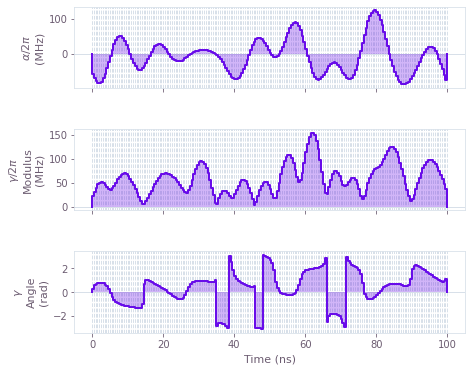# How to perform model-based optimization with basis functions

Create optimized controls from a user-defined set of basis functions

The Q-CTRL Python package exposes a highly-flexible optimization engine for general-purpose gradient-based optimization. In chopped random basis (CRAB) optimization, pulses are defined via optimizable linear combinations from a set of basis functions, which can greatly reduce the dimensionality of the optimization search space. Traditionally, a randomized Fourier basis is used, although the same technique has also seen success with other bases, for example Slepian functions.

## Summary workflow

### 1. Define basis function for signal composition in the graph

The Q-CTRL optimization engine provides a convenience function, qctrl.operations.real_fourier_pwc_signal, for creating optimizable signals in a Fourier basis, suitable for use in a CRAB optimization. Other bases are supported by the framework, but require the user to manually provide operations that compute the appropriate linear combinations.

### 2. Execute graph-based optimization

With the graph object created, an optimization can be run using the qctrl.functions.calculate_optimization function. The cost, the outputs, and the graph must be provided. The function returns the results of the optimization.

## Worked example: CRAB optimization on a qutrit

In this example, we perform a CRAB optimization (in the Fourier basis) of a qutrit system in which we effect a single-qubit gate while minimizing leakage out of the computational subspace. The system is described by the following Hamiltonian:

\begin{align*} H(t) = & \frac{\chi}{2} (a^\dagger)^2 a^2 + \gamma(t) (1+\beta(t))a + \gamma^*(t)(1+\beta(t)) a^\dagger + \frac{\alpha(t)}{2} a^\dagger a \,, \end{align*}

where $\chi$ is the anharmonicity, $\gamma(t)$ and $\alpha(t)$ are, respectively, complex and real time-dependent pulses, $\beta$ is a small, slowly-varying stochastic amplitude noise process, and $a = |0 \rangle \langle 1 | + \sqrt{2} |1 \rangle \langle 2 |$.

import matplotlib.pyplot as plt
import numpy as np
from qctrlvisualizer import get_qctrl_style, plot_controls

from qctrl import Qctrl

plt.style.use(get_qctrl_style())

# Starting a session with the API
qctrl = Qctrl()

# Define standard matrices
a = np.array([[0.0, 1.0, 0.0], [0.0, 0.0, np.sqrt(2)], [0.0, 0.0, 0.0]], dtype=complex)
ad2a2 = np.matmul(np.matmul(a.T, a.T), np.matmul(a, a))
[[1.0, 1.0, 0], [1.0, -1.0, 0], [0, 0, np.sqrt(2)]], dtype=complex
) / np.sqrt(2)
qubit_projector = np.array([[1, 0, 0], [0, 1, 0], [0, 0, 0]], dtype=complex)

# Define physical constraints
chi = 2 * np.pi * -300.0 * 1e6  # Hz
gamma_max = 2 * np.pi * 30e6  # Hz
alpha_max = 2 * np.pi * 30e6  # Hz
segment_count = 200
duration = 100e-9  # s

# Create graph object
with qctrl.create_graph() as graph:
# Create gamma(t) signal in Fourier bases. To demonstrate the full
# flexibility, we show how to use both randomized and optimizable
# basis elements. Elements with fixed frequencies may be chosen too.
gamma_i = qctrl.operations.real_fourier_pwc_signal(
duration=duration, segment_count=segment_count, randomized_frequency_count=10
)
gamma_q = qctrl.operations.real_fourier_pwc_signal(
duration=duration, segment_count=segment_count, optimizable_frequency_count=10
)
gamma = qctrl.operations.complex_value(
gamma_i * gamma_max, gamma_q * gamma_max, name="gamma"
)

# Create alpha(t) signal
alpha = qctrl.operations.real_fourier_pwc_signal(
duration=duration,
segment_count=segment_count,
initial_coefficient_lower_bound=-alpha_max,
initial_coefficient_upper_bound=alpha_max,
optimizable_frequency_count=10,
name="alpha",
)

# Create anharmonicity term
anharmonicity = ad2a2 * chi / 2

# Create drive term
drive = qctrl.operations.pwc_operator_hermitian_part(2 * gamma * a)

# Create clock shift term
shift = alpha * ada / 2

# Create infidelity (note that we project the target operator to the
# qubit subspace in order to calculate infidelity only in the subspace,
# and set a filter function projector in order to evaluate robustness
# only from the subspace).
target_operator = qctrl.operations.target(
)

infidelity = qctrl.operations.infidelity_pwc(
hamiltonian=anharmonicity + drive + shift,
target=target_operator,
noise_operators=[drive],
name="infidelity",
)

# Run the optimization
optimization_result = qctrl.functions.calculate_optimization(
cost_node_name="infidelity", output_node_names=["alpha", "gamma"], graph=graph
)

print(f"\nOptimized cost:\t{optimization_result.cost:.3e}")

# Plot the optimized controls
plot_controls(
plt.figure(),
controls={
"$\\alpha$": optimization_result.output["alpha"],
"$\\gamma$": optimization_result.output["gamma"],
},
)
plt.show()

Your task calculate_optimization has started.